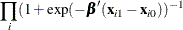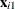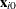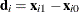### Example 54.11 Conditional Logistic Regression for Matched Pairs Data

In matched pairs, or case-control, studies, conditional logistic regression is used to investigate the relationship between an outcome of being an event (case) or a nonevent (control) and a set of prognostic factors.

The following data are a subset of the data from the Los Angeles Study of the Endometrial Cancer Data in Breslow and Day (1980). There are 63 matched pairs, each consisting of a case of endometrial cancer (`Outcome`=1) and a control (`Outcome`=0). The case and corresponding control have the same `ID`. Two prognostic factors are included: `Gall` (an indicator variable for gall bladder disease) and `Hyper` (an indicator variable for hypertension). The goal of the case-control analysis is to determine the relative risk for gall bladder disease, controlling for the effect of hypertension.

```data Data1;
do ID=1 to 63;
do Outcome = 1 to 0 by -1;
input Gall Hyper @@;
output;
end;
end;
datalines;
0 0  0 0    0 0  0 0    0 1  0 1    0 0  1 0    1 0  0 1
0 1  0 0    1 0  0 0    1 1  0 1    0 0  0 0    0 0  0 0
1 0  0 0    0 0  0 1    1 0  0 1    1 0  1 0    1 0  0 1
0 1  0 0    0 0  1 1    0 0  1 1    0 0  0 1    0 1  0 0
0 0  1 1    0 1  0 1    0 1  0 0    0 0  0 0    0 0  0 0
0 0  0 1    1 0  0 1    0 0  0 1    1 0  0 0    0 1  0 0
0 1  0 0    0 1  0 0    0 1  0 0    0 0  0 0    1 1  1 1
0 0  0 1    0 1  0 0    0 1  0 1    0 1  0 1    0 1  0 0
0 0  0 0    0 1  1 0    0 0  0 1    0 0  0 0    1 0  0 0
0 0  0 0    1 1  0 0    0 1  0 0    0 0  0 0    0 1  0 1
0 0  0 0    0 1  0 1    0 1  0 0    0 1  0 0    1 0  0 0
0 0  0 0    1 1  1 0    0 0  0 0    0 0  0 0    1 1  0 0
1 0  1 0    0 1  0 0    1 0  0 0
;
```

There are several ways to approach this problem with PROC LOGISTIC:

SAS statements and selected results for these four approaches are given in the remainder of this example.

#### Conditional Analysis Using the STRATA Statement

In the following statements, PROC LOGISTIC is invoked with the `ID` variable declared in the STRATA statement to obtain the conditional logistic model estimates for a model containing `Gall` as the only predictor variable:

```proc logistic data=Data1;
strata ID;
model outcome(event='1')=Gall;
run;
```

Results from the conditional logistic analysis are shown in Output 54.11.1. Note that there is no intercept term in the Analysis of Maximum Likelihood Estimates tables.

The odds ratio estimate for `Gall` is 2.60, which is marginally significant (p = 0.0694) and which is an estimate of the relative risk for gall bladder disease. A 95% confidence interval for this relative risk is (0.927, 7.293).

Output 54.11.1: Conditional Logistic Regression (Gall as Risk Factor)

The LOGISTIC Procedure

Conditional Analysis

Model Information
Data Set WORK.DATA1
Response Variable Outcome
Number of Response Levels 2
Number of Strata 63
Model binary logit
Optimization Technique Newton-Raphson ridge

 Number of Observations Read 126 126

Response Profile
Ordered
Value
Outcome Total
Frequency
1 0 63
2 1 63

Probability modeled is Outcome=1.

Strata Summary
Response
Pattern
Outcome Number of
Strata
Frequency
0 1
1 1 1 63 126

Newton-Raphson Ridge Optimization

Without Parameter Scaling

 Convergence criterion (GCONV=1E-8) satisfied.

Model Fit Statistics
Criterion Without Covariates With Covariates
AIC 87.337 85.654
SC 87.337 88.490
-2 Log L 87.337 83.654

Testing Global Null Hypothesis: BETA=0
Test Chi-Square DF Pr > ChiSq
Likelihood Ratio 3.6830 1 0.0550
Score 3.5556 1 0.0593
Wald 3.2970 1 0.0694

Analysis of Conditional Maximum Likelihood Estimates
Parameter DF Estimate Standard
Error
Wald
Chi-Square
Pr > ChiSq
Gall 1 0.9555 0.5262 3.2970 0.0694

Odds Ratio Estimates
Effect Point Estimate 95% Wald
Confidence Limits
Gall 2.600 0.927 7.293

#### Exact Analysis Using the STRATA Statement

When you believe there are not enough data or that the data are too sparse, you can perform a stratified exact logistic regression. The following statements perform stratified exact logistic regressions on the original data set by specifying both the STRATA and EXACT statements:

```proc logistic data=Data1 exactonly;
strata ID;
model outcome(event='1')=Gall;
exact Gall / estimate=both;
run;
```

Output 54.11.2: Exact Logistic Regression (Gall as Risk Factor)

The LOGISTIC Procedure

Exact Conditional Analysis

Exact Conditional Tests
Effect Test Statistic p-Value
Exact Mid
Gall Score 3.5556 0.0963 0.0799
Probability 0.0327 0.0963 0.0799

Exact Parameter Estimates
Parameter Estimate Standard Error 95% Confidence Limits Two-sided p-Value
Gall 0.9555 0.5262 -0.1394 2.2316 0.0963

Exact Odds Ratios
Parameter Estimate 95% Confidence Limits Two-sided p-Value
Gall 2.600 0.870 9.315 0.0963

Note that the score statistic in the Conditional Exact Tests table in Output 54.11.2 is identical to the score statistic in Output 54.11.1 from the conditional analysis. The exact odds ratio confidence interval is much wider than its conditional analysis counterpart, but the parameter estimates are similar. The exact analysis confirms the marginal significance of `Gall` as a predictor variable.

#### Conditional Analysis Using Transformed Data

When each matched set consists of one event and one nonevent, the conditional likelihood is given bywhereandare vectors representing the prognostic factors for the event and nonevent, respectively, of the ith matched set. This likelihood is identical to the likelihood of fitting a logistic regression model to a set of data with constant response, where the model contains no intercept term and has explanatory variables given by(Breslow, 1982).

To apply this method, the following DATA step transforms each matched pair into a single observation, where the variables `Gall` and `Hyper` contain the differences between the corresponding values for the case and the control (case–control). The variable `Outcome`, which will be used as the response variable in the logistic regression model, is given a constant value of 0 (which is the `Outcome` value for the control, although any constant, numeric or character, will suffice).

```data Data2;
set Data1;
drop id1 gall1 hyper1;
retain id1 gall1 hyper1 0;
if (ID = id1) then do;
Gall=gall1-Gall; Hyper=hyper1-Hyper;
output;
end;
else do;
id1=ID; gall1=Gall; hyper1=Hyper;
end;
run;
```

Note that there are 63 observations in the data set, one for each matched pair. Since the number of observations n is halved, statistics that depend on n such as R Square (see the Generalized Coefficient of Determination section) will be incorrect. The variable `Outcome` has a constant value of 0.

In the following statements, PROC LOGISTIC is invoked with the NOINT option to obtain the conditional logistic model estimates. Because the option CLODDS=PL is specified, PROC LOGISTIC computes a 95% profile-likelihood confidence interval for the odds ratio for each predictor variable; note that profile-likelihood confidence intervals are not currently available when a STRATA statement is specified.

```proc logistic data=Data2;
model outcome=Gall / noint clodds=PL;
run;
```

The results are not displayed here.

#### Exact Analysis Using Transformed Data

Sometimes the original data set in a matched-pairs study is too large for the exact methods to handle. In such cases it might be possible to use the transformed data set. The following statements perform exact logistic regressions on the transformed data set. The results are not displayed here.

```proc logistic data=Data2 exactonly;
model outcome=Gall / noint;
exact Gall / estimate=both;
run;
```